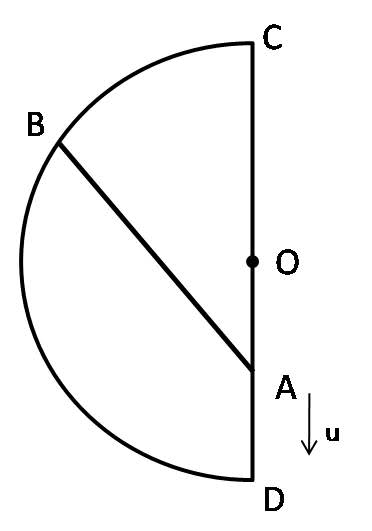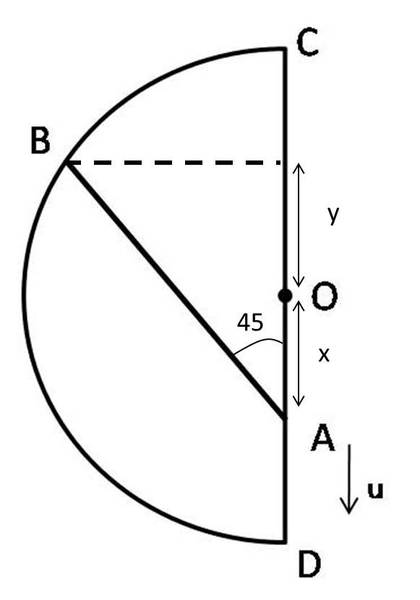# Beads on Semi-Circular wire

## Homework Statement

Two beads A and B move along a semicircular wire frame as shown in the figure as shown in figure. The beads are connected by an inelastic string which always remains tight. At an instant the speed of A is u, angle BAC is 45 degrees and angle BOC is 75 degrees, where O is the centre of semicircular arc. The speed of bead B at that instant is.## The Attempt at a Solution

I really have no idea on how to begin with this. How should i go on forming equations?
Please point me in the right direction.

Thanks!

## Answers and Replies

Hi again :tongue2:

Since the string is not extensible, the vertical velocity of B will always have to be equal to the velocity of A. Can you frame an equation for this?

Hi again :tongue2:

Since the string is not extensible, the vertical velocity of B will always have to be equal to the velocity of A. Can you frame an equation for this?

I did that once but i thought i am trying my own foolish things.
I assumed that at any instant the distance of A from O is x and that of B is y.
l=(x+y)cos45
Differentiating with respect to time, i get:
(dy/dt)=-(dx/dt)

How can i proceed further?

l=(x+y)cos45

Umm noo..how did you get that?

You can simply equate the vertical velocity component of B to u. The velocity of B will be have its direction tangential to the ring...what does this tell you about its vertical component?

TSny
Homework Helper
Gold Member
Hi again :tongue2:

Since the string is not extensible, the vertical velocity of B will always have to be equal to the velocity of A. Can you frame an equation for this?

Hello, Infinitum. I don't see how your statement can be true. If the vertical component of velocity of B equals the velocity of A, then during a time Δt, both particles would move the same distance vertically. But B will also move horizontally during this time. So, it seems to me that this would change the distance between A and B.

TSny
Homework Helper
Gold Member

## The Attempt at a Solution

I really have no idea on how to begin with this. How should i go on forming equations?
Please point me in the right direction.

You might give us some context for the problem since there are different methods for solving it. If it's a "related rates problem" from a calculus course then you would be expected to use calculus. Or if it's a problem where you can use any method you want, then you can solve it with just geometry, trig, and some insight.

Umm noo..how did you get that?

You can simply equate the vertical velocity component of B to u. The velocity of B will be have its direction tangential to the ring...what does this tell you about its vertical component?

Sorry, i think i did not explain it correctly. My assumption can be better shown by this pic:So what i did was incorrect? and why?

You might give us some context for the problem since there are different methods for solving it. If it's a "related rates problem" from a calculus course then you would be expected to use calculus. Or if it's a problem where you can use any method you want, then you can solve it with just geometry, trig, and some insight.

Well, it isn't specified that i need to do the problem by a specified method, any method will do.

TSny said:
...you can solve it with just geometry, trig, and some insight.
Would you be so kind to tell me how i can solve by this? :tongue2:

ehild
Homework Helper
Hi Pranav,

The angles change with time so just call them α and β or anything else. Find relation between them and x. You know that u=dx/dt. The speed of the other bead on the circle is v=R|dβ/dt|. You need to differentiate, using that the length of the cord is constant in time, and so is the radius of the circle.

ehild

#### Attachments

Hi Pranav,

The angles change with time so just call them α and β or anything else. Find relation between them and x. You know that u=dx/dt. The speed of the other bead on the circle is v=R|dβ/dt|. You need to differentiate, using that the length of the cord is constant in time, and so is the radius of the circle.

ehild

Hello ehild!As you said i tried finding some relations,
Ist relation, Lsin(α)=Rsin(β)=d
IInd Relation, Lcos(α)-Rcos(β)=x
Differentiating the second relation with respect to time,

Rsin(β)(dβ/dt)-Lsin(α)(dα/dt)=dx/dt
I can use the first relation here but i don't seem to reach the answer.

Instead of using law of sines, why not try using law of cosines.

Last edited:
Hello, Infinitum. I don't see how your statement can be true. If the vertical component of velocity of B equals the velocity of A, then during a time Δt, both particles would move the same distance vertically. But B will also move horizontally during this time. So, it seems to me that this would change the distance between A and B.

Hi TSny. You are right, I meant to write that the velocity component of A along the length of the rod has to be equal to the velocity component of B along the length of the rod, so that the rod length remains the same.

Sorry, i think i did not explain it correctly. My assumption can be better shown by this pic:So what i did was incorrect? and why?

Oh, I see. You had mentioned that the distance of B from O is y earlier, that led me into confusion :tongue2:

I also made a little mistake while saying their vertical components are equal. Instead, their velocities along the rod have to be the same, for no extension.

Oh, I see. You had mentioned that the distance of B from O is y earlier, that led me into confusion :tongue2:

I also made a little mistake while saying their vertical components are equal. Instead, their velocities along the rod have to be the same, for no extension.

Umm..so what i did is incorrect?

How should i proceed now?

Umm..so what i did is incorrect?

Yes, you got "l=(x+y)cos45"

But it should be $lcos45 = x+y$

And you cannot simply differentiate this because the angle is changing continuously, as ehild indicated.

How should i proceed now?

An easier approach is equating the velocities of A and B along the rod. Let the velocity of B be v, and then try to solve the problem.

An easier approach is equating the velocities of A and B along the rod. Let the velocity of B be v, and then try to solve the problem.

Oh, that's a nice hint, thanks for the help, i got the answer.Oh, that's a nice hint, thanks for the help, i got the answer.Way to go!TSny
Homework Helper
Gold Member
Hi TSny. You are right, I meant to write that the velocity component of A along the length of the rod has to be equal to the velocity component of B along the length of the rod, so that the rod length remains the same.

That's a very nice way to solve it.

I had solved it by setting up a velocity triangle based on

VB = VA + VB/A . (vector addition!)

where VB/A is the velcity of B relative to A. VB/A must be perpendicular to line AB in order for AB to keep a fixed distance apart. This is similar to your statement that the velocity components of A and B along line AB must be equal. From the velocity triangle you can then use the law of sines to get the result.

But I like your approach much better. It gets the answer right away.

Thanks.

ehild
Homework Helper
An easier approach is equating the velocities of A and B along the rod. Let the velocity of B be v, and then try to solve the problem.

It is ingenious, Infinitum!

My method is much more complicated.
The speed of the bead on the vertical line is dx/dt=u.
The speed of the bead on the circle is v=Rdβ/dt=Rω

From the cosine law in the tringle AOB,

$$L^2=R^2+x^2+2Rxcos(\beta)$$

With implicit differentiation,

$$0=x u+R u cos(\beta)-Rx\sin(\beta) ω$$

isolating v=Rω

$$v=u\frac{(1+\frac{R}{x}cos(\beta))}{\sin(\beta)}$$

Form the sine law, $$\frac{R}{x}=\frac{\sin(\alpha)}{\sin(\beta-\alpha)}$$

Plugging in and simplifying, we get:

$$v=u\frac{cos(\alpha)}{\sin(\beta-\alpha)}$$

ehild

#### Attachments

The speed of the bead on the vertical line is dx/dt=u.
The speed of the bead on the circle is v=Rdβ/dt=Rω

From the cosine law in the tringle AOB,

$$L^2=R^2+x^2+2Rxcos(\beta)$$

With implicit differentiation,

$$0=x u+R u cos(\beta)-Rx\sin(\beta) ω$$

isolating v=Rω

$$v=u\frac{(1+\frac{R}{x}cos(\beta))}{\sin(\beta)}$$

Form the sine law, $$\frac{R}{x}=\frac{\sin(\alpha)}{\sin(\beta-\alpha)}$$

Plugging in and simplifying, we get:

$$v=u\frac{cos(\alpha)}{\sin(\beta-\alpha)}$$

ehild
It's really complicated. :tongue:
Thanks for an alternative solution.